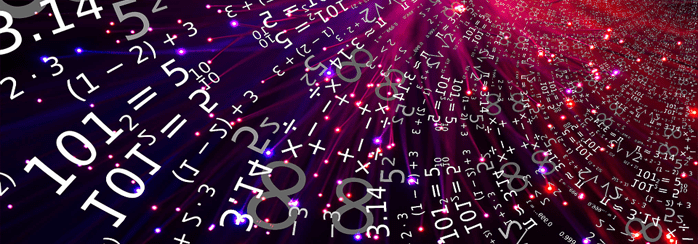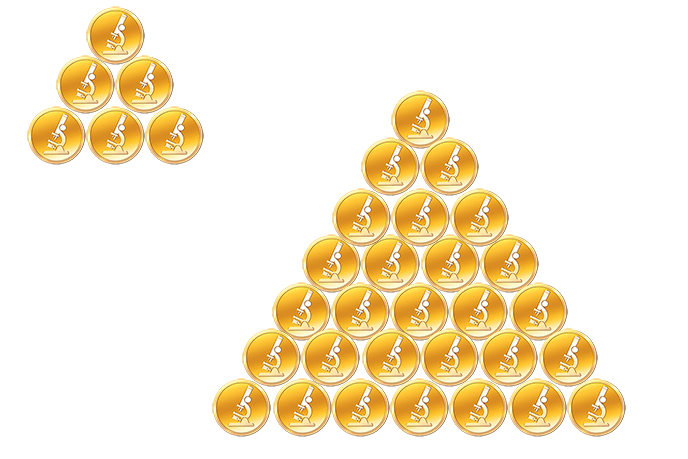# The Secret of Perfect NumbersNumbers accompany us from birth until the last days and we can’t imagine the life without them. Among all the numbers, there are some special ones, named perfect. And despite a lot of papers are devoted to these perfect numbers, only 24 such numbers have been determined so far. So what is special about these numbers? Well, the number is called perfect if it is equal to the sum of all its proper divisors excluding itself. Now, let’s find out more!

The first perfect number is the number $6$ because its divisors are $1,2,3,6$ and $6 = 1 + 2 + 3$. The second perfect number is $28$. The dividers of $28$ are such numbers as $1,2,4,7,14,28$ and indeed $1 + 2 + 4 + 7 + 14 = 28$. The first two perfect numbers were known in antiquity. The next two numbers $496$ and $8128$ were found by Euclid. Moreover, he gave the key to the search of all even perfect numbers: if the number $p = 1 + 2 + 4 + … + 2n = 2n + 1-1$ is the prime, then the number $2nr$ is the perfect one. The correctness of this statement can be proven by considering all the proper divisors of $2nr$: $1,2,4, …, 2n, 2n + 1-1,2 (2n + 1-1), 4 (2n + 1-1), …, 2n-1 (2n + 1-1)$ and calculating their sum. All perfect numbers, which can be found by the Euclid rule, are even.

The beauty of the perfect numbers can be seen in their properties:

• All even perfect numbers except $6$ are the sum of the cubes of consecutive odd natural numbers:

$28 = 1^3 + 3^3$;

$496 = 1^3 + 3^3+ 5^3 + 7^3$;

$8 128 = 1^3 + 3^3+ 5^3 + 7^3 + 9^3 + 11^3 + 13^3 + 15^3$.

• All even perfect numbers are triangular numbers. This means that taking the perfect number of coins of the same size, we can always lay them down into the equilateral triangle:• All even perfect numbers are hexagonal and hence can be represented as $n · (2n-1)$ for some natural number $n$:

$6 = 2 · 3$, $n = 2$;
$28 = 4 · 7$, $n = 4$;
$496 = 16 · 31$, $n = 16$;
$8 128 = 64 · 127$, $n = 64$.

• The sum of all the numbers, which are back dividers to the perfect number equals 2. This is a direct consequence of the perfect numbers definition. It is easily seen by multiplying both sides of each following equation by the corresponding perfect number and reducing fractions on the left side:

$\frac{1}{1} + \frac{1}{2} + \frac{1}{3} + \frac{1}{6} = 2$;
$\frac{1}{1} + \frac{1}{2} + \frac{1}{4} + \frac{1}{7} + \frac{1}{1}4 + \frac{1}{28} = 2$;
$\frac{1}{1} + \frac{1}{2} + \frac{1}{4} + \frac{1}{8} + \frac{1}{16} + \frac{1}{31} + \frac{1}{62} + \frac{1}{124} + \frac{1}{248} + \frac{1}{496} = 2$;
$\frac{1}{1} + \frac{1}{2} + \frac{1}{4} + \frac{1}{8} + \frac{1}{16} +\frac{1}{32} + \frac{1}{64} + \frac{1}{127} + \frac{1}{254} + \frac{1}{508} + \frac{1}{1016} + \frac{1}{2032} + \frac{1}{4064} + \frac{1}{8128} = 2$.

• All even perfect numbers in binary notation contain first $p$ units, followed by $p – 1$ zeros:

$6 = 110_2$   $p = 2$;
$28 = 11100_2$   $p = 3$;
$496 = 111110000_2$   $p = 5$;
$8128 = 1111111000000_2$   $p = 7$.

The remainder of the even perfect number division by 9 (except 6) is equal to 1. As a consequence, the sum of digits of the even perfect numbers (except 6) equals 1:

$2 + 8 = 10$,   $1 + 0 = 1$;
$4 + 9 + 6 = 19$,   $1 + 9 = 10$,   $1 + 0 = 1$;
$8 + 1 + 2 + 8 = 19$,   $1 + 9 = 10$,   $1 + 0 = 1$;
$3 + 3 + 5 + 5 + 0 + 3 + 3 + 6 = 28$,   $2 + 8 = 10$,   $1 + 0 = 1$.Note that it is still unknown whether the odd perfect numbers exist and if it is possible to find the largest even perfect number. As the contemporary mathematicians say, “the works devoted to odd perfect numbers remind the hunt for a ghost, which no one had seen, but there are many indigenous studies on how it cannot look like.” Maybe, the future generation will do their best and prove the existence of odd perfect numbers.

4 Shares
Filed under Math.